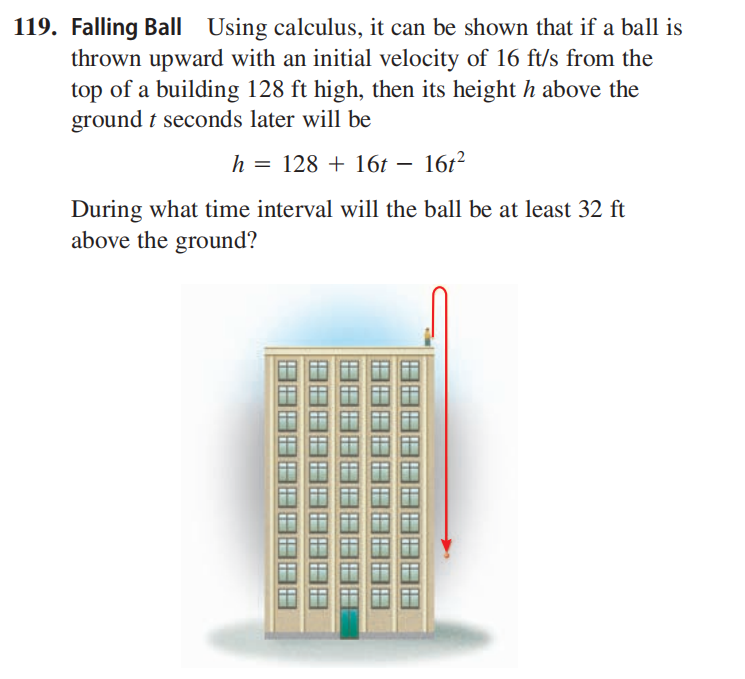### ¿Todavía tienes preguntas de matemáticas?

Pregunte a nuestros tutores expertos
Algebra
Pregunta119. Falling Ball Using calculus, it can be shown that if a ball is thrown upward with an initial velocity of $$16 ft / s$$ from the top of a building $$128 ft$$ high, then its height $$h$$ above the ground $$t$$ seconds later will be

$$h = 128 + 16 t - 16 t ^ { 2 }$$

$$\text { 119. From } 0 \text { s to } 3 s$$